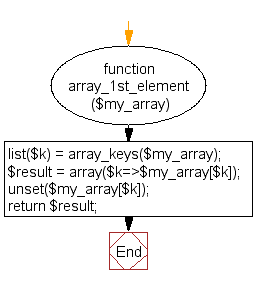﻿ PHP Array Exercise: Get an array with the first key and value - w3resource# PHP Array Exercises : Get an array with the first key and value

## PHP Array: Exercise-47 with Solution

Write a PHP function to get an array with the first key and value.

Sample Solution:

PHP Code:

``````<?php
function array_1st_element(\$my_array)
{
list(\$k) = array_keys(\$my_array);
\$result  = array(\$k=>\$my_array[\$k]);
unset(\$my_array[\$k]);
return \$result;
}
\$colors = array('c1'=>'Red','c2'=>'Green','c3'=>'Black');
print_r(array_1st_element(\$colors));
?>
```
```

Sample Output:

```Array
(
[c1] => Red
)
```

Flowchart:PHP Code Editor:

What is the difficulty level of this exercise?

﻿

## PHP: Tips of the Day

PHP: Extract numbers from a string

```\$str = 'In My Cart : 11 12 items';
preg_match_all('!\d+!', \$str, \$matches);
print_r(\$matches);
```